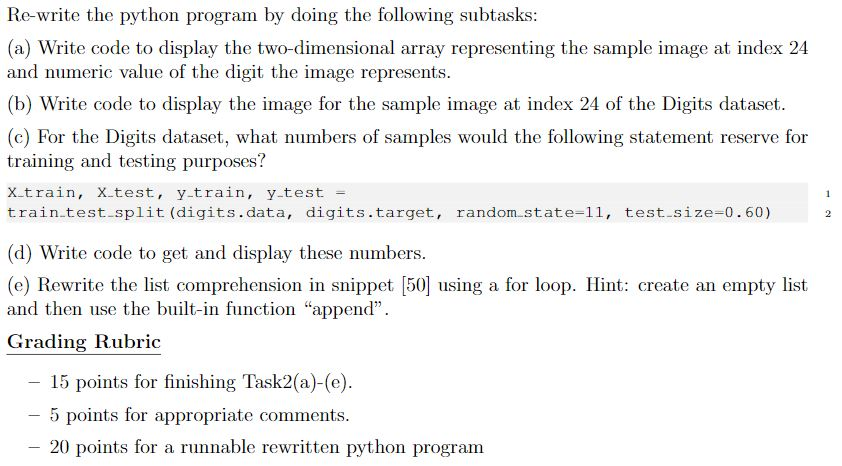# (Solved) : Usr Bin Env Python Coding Utf 8 36 Sklearndatasets Import Loaddigits 37 Digits Loaddigits Q42764770 . . .#!/usr/bin/env python
# coding: utf-8

# In:

# In:

# In:

print(digits.DESCR)

# In:

digits.target[::100] # target values of every 100th sample

# In:

digits.images # show array for sample image at index 13

# In:

import matplotlib.pyplot as plt

# In:

plt.imshow(digits.images)

# In:

figure, axes = plt.subplots(nrows=4, ncols=6, figsize=(6, 4))

for item in zip(axes.ravel(), digits.images,digits.target):
axes, image, target = item
axes.imshow(image, cmap=plt.cm.gray_r)
axes.set_xticks([]) # remove x-axis tick marks
axes.set_yticks([]) # remove y-axis tick marks
axes.set_title(target)
plt.tight_layout()

# In:

from sklearn.model_selection import train_test_split
X_train, X_test, y_train, y_test = train_test_split(
digits.data, digits.target, random_state=11, test_size=0.20)
# random_state for reproducibility

# In:

X_train.shape

# In:

from sklearn.neighbors import KNeighborsClassifier
knn = KNeighborsClassifier()
knn.fit(X=X_train, y=y_train)

# In:

predicted = knn.predict(X=X_test)
expected = y_test

predicted[:20]
expected[:20]

# In:

predicted[:20]

# In:

expected[:20]

# In:

wrong = [(p, e) for (p, e) in zip(predicted, expected) if p !=e]

# In:

wrong

# In:

print(“Model accuracy:%.2f%%” %(knn.score(X_test,y_test)*100))

# In[ ]:

# In[ ]:

Re-write the python program by doing the following subtasks: (a) Write code to display the two-dimensional array representing the sample image at index 24 and numeric value of the digit the image represents (b) Write code to display the image for the sample image at index 24 of the Digits dataset (c) For the Digits dataset, what numbers of samples would the following statement reserve for training and testing purposes? X train, Xtest, y.train, y test train.test split (digits.data, digits.target, random state – 11, test size-0.60) 1 2 (d) Write code to get and display these numbers (e) Rewrite the list comprehension in snippet  using a for loop. Hint: create an empty list and then use the built-in function “append” Grading Rubric 15 points for finishing Task2(a)-(e 5 points for appropriate comments – 20 points for a runnable rewritten python program Show transcribed image text Re-write the python program by doing the following subtasks: (a) Write code to display the two-dimensional array representing the sample image at index 24 and numeric value of the digit the image represents (b) Write code to display the image for the sample image at index 24 of the Digits dataset (c) For the Digits dataset, what numbers of samples would the following statement reserve for training and testing purposes? X train, Xtest, y.train, y test train.test split (digits.data, digits.target, random state – 11, test size-0.60) 1 2 (d) Write code to get and display these numbers (e) Rewrite the list comprehension in snippet  using a for loop. Hint: create an empty list and then use the built-in function “append” Grading Rubric 15 points for finishing Task2(a)-(e 5 points for appropriate comments – 20 points for a runnable rewritten python program

Answer to #!/usr/bin/env python # coding: utf-8 # In: from sklearn.datasets import load_digits # In: digits = load_digits(…

We are the best freelance writing portal. Looking for online writing, editing or proofreading jobs? We have plenty of writing assignments to handle.